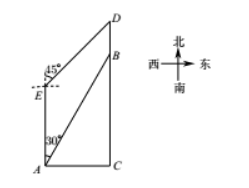(1) 求步道 $D E$ 的长度 (精确到个位)：
(2) 点 $D$ 处有直饮水, 小红从 $A$ 出发沿人行步道去取水, 可以经过点 $B$ 到达点 $D$, 也可以经过点 $E$ 到达点 D. 请计算说明他走哪一条路较近?
(参考数据: $\sqrt{2} \approx 1.414, \sqrt{3} \approx 1.732$ )①点击 首页查看更多试卷和试题 , 点击查看 本题所在试卷